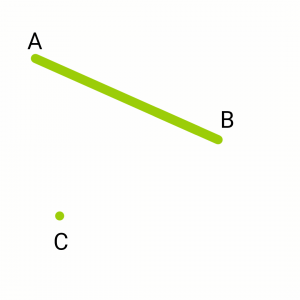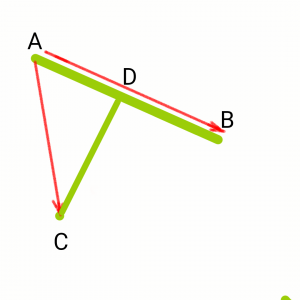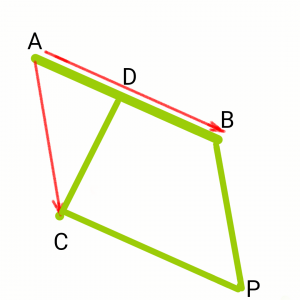# Shortest distance between a Line and a Point in a 3-D plane

Given a line passing through two points A and B and an arbitrary point C in a 3-D plane, the task is to find the shortest distance between the point C and the line passing through the points A and B.

Examples:

```Input: A = (5, 2, 1), B = (3, 1, -1), C = (0, 2, 3)
Output: Shortest Distance is 5

Input: A = (4, 2, 1), B = (3, 2, 1), C = (0, 2, 0)
Output: Shortest Distance is 1
```

Consider a point C and a line that passes through A and B as shown in the below figure.Now Consider the vectors, AB and AC and the shortest distance as CD. The Shortest Distance is always the perpendicular distance. The point D is taken on AB such that CD is perpendicular to AB.Construct BP and CP as shown in the figure to form a Parallelogram. Now C is a vertex of parallelogram ABPC and CD is perpendicular to Side AB. Hence CD is the height of the parallelogram.Note: In the case when D does not fall on line segment AB there will be a point D’ such that PD’ is perpendicular to AB and D’ lies on line segment AB with CD = PD’.

The magnitude of cross product AB and AC gives the Area of the parallelogram. Also, the area of a parallelogram is Base * Height = AB * CD. So,

`CD = |ABxAC| / |AB|`

Below is the CPP program to find the shortest distance:

 `// C++ program to find the Shortest ` `// Distance Between A line and a ` `// Given point. ` `#include ` `using` `namespace` `std; ` ` `  `class` `Vector { ` `private``: ` `    ``int` `x, y, z; ` `    ``// 3D Coordinates of the Vector ` ` `  `public``: ` `    ``Vector(``int` `x, ``int` `y, ``int` `z) ` `    ``{ ` `        ``// Constructor ` `        ``this``->x = x; ` `        ``this``->y = y; ` `        ``this``->z = z; ` `    ``} ` `    ``Vector operator+(Vector v); ``// ADD 2 Vectors ` `    ``Vector operator-(Vector v); ``// Subtraction ` `    ``int` `operator^(Vector v); ``// Dot Product ` `    ``Vector operator*(Vector v); ``// Cross Product ` `    ``float` `magnitude() ` `    ``{ ` `        ``return` `sqrt``(``pow``(x, 2) + ``pow``(y, 2) + ``pow``(z, 2)); ` `    ``} ` `    ``friend` `ostream& operator<<(ostream& out, ``const` `Vector& v); ` `    ``// To output the Vector ` `}; ` ` `  `// ADD 2 Vectors ` `Vector Vector::operator+(Vector v) ` `{ ` `    ``int` `x1, y1, z1; ` `    ``x1 = x + v.x; ` `    ``y1 = y + v.y; ` `    ``z1 = z + v.z; ` `    ``return` `Vector(x1, y1, z1); ` `} ` ` `  `// Subtract 2 vectors ` `Vector Vector::operator-(Vector v) ` `{ ` `    ``int` `x1, y1, z1; ` `    ``x1 = x - v.x; ` `    ``y1 = y - v.y; ` `    ``z1 = z - v.z; ` `    ``return` `Vector(x1, y1, z1); ` `} ` ` `  `// Dot product of 2 vectors ` `int` `Vector::operator^(Vector v) ` `{ ` `    ``int` `x1, y1, z1; ` `    ``x1 = x * v.x; ` `    ``y1 = y * v.y; ` `    ``z1 = z * v.z; ` `    ``return` `(x1 + y1 + z1); ` `} ` ` `  `// Cross product of 2 vectors ` `Vector Vector::operator*(Vector v) ` `{ ` `    ``int` `x1, y1, z1; ` `    ``x1 = y * v.z - z * v.y; ` `    ``y1 = z * v.x - x * v.z; ` `    ``z1 = x * v.y - y * v.x; ` `    ``return` `Vector(x1, y1, z1); ` `} ` ` `  `// Display Vector ` `ostream& operator<<(ostream& out, ` `                    ``const` `Vector& v) ` `{ ` `    ``out << v.x << ``"i "``; ` `    ``if` `(v.y >= 0) ` `        ``out << ``"+ "``; ` `    ``out << v.y << ``"j "``; ` `    ``if` `(v.z >= 0) ` `        ``out << ``"+ "``; ` `    ``out << v.z << ``"k"` `<< endl; ` `    ``return` `out; ` `} ` ` `  `// calculate shortest dist. from point to line ` `float` `shortDistance(Vector line_point1, Vector line_point2, ` `                    ``Vector point) ` `{ ` `    ``Vector AB = line_point2 - line_point1; ` `    ``Vector AC = point - line_point1; ` `    ``float` `area = Vector(AB * AC).magnitude(); ` `    ``float` `CD = area / AB.magnitude(); ` `    ``return` `CD; ` `} ` ` `  `// Driver program ` `int` `main() ` `{ ` `    ``// Taking point C as (2, 2, 2) ` `    ``// Line Passes through A(4, 2, 1) ` `    ``// and B(8, 4, 2). ` `    ``Vector line_point1(4, 2, 1), line_point2(8, 4, 2); ` `    ``Vector point(2, 2, 2); ` ` `  `    ``cout << ``"Shortest Distance is : "` `         ``<< shortDistance(line_point1, line_point2, point); ` ` `  `  ``return` `0; ` `} `

Output:

```Shortest Distance is : 1.63299
```

Attention reader! Don’t stop learning now. Get hold of all the important DSA concepts with the DSA Self Paced Course at a student-friendly price and become industry ready.

My Personal Notes arrow_drop_upFinal year BTech IT student at DTU, Upcoming Technology Analyst at Morgan Stanley

If you like GeeksforGeeks and would like to contribute, you can also write an article using contribute.geeksforgeeks.org or mail your article to contribute@geeksforgeeks.org. See your article appearing on the GeeksforGeeks main page and help other Geeks.

Please Improve this article if you find anything incorrect by clicking on the "Improve Article" button below.